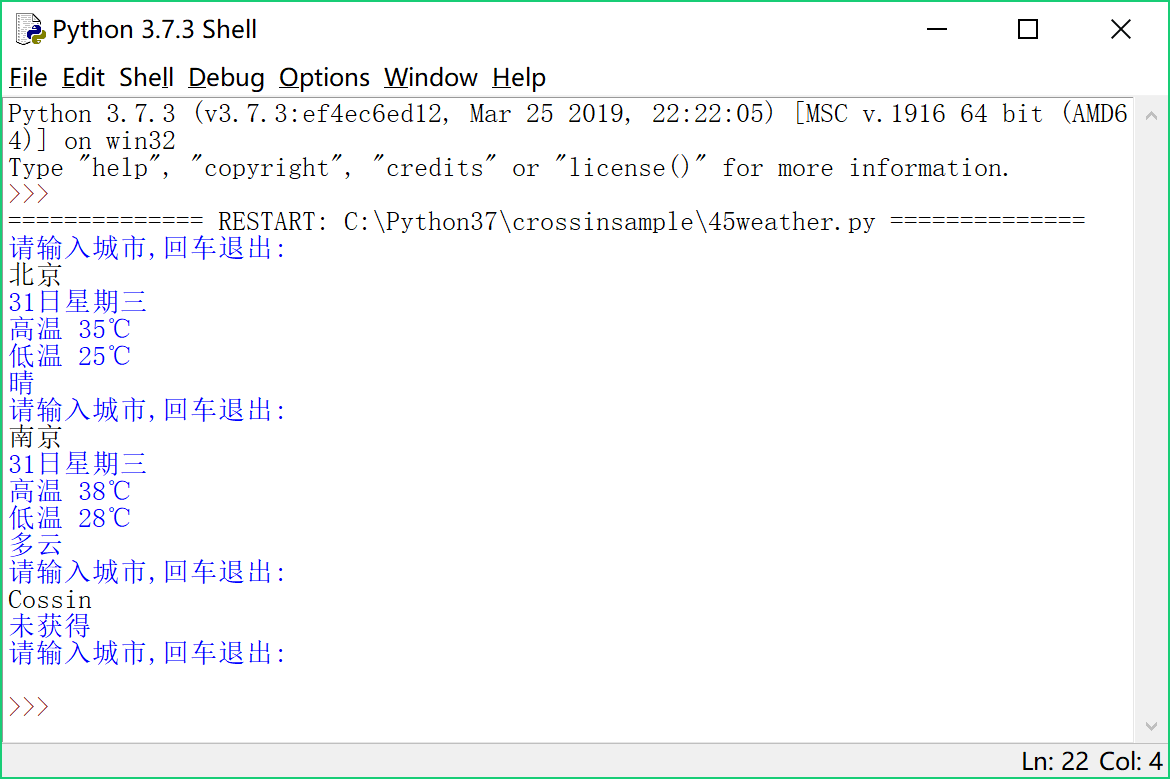## 【Python 第45课】查天气（3）

{

"data":{

"yesterday":{"date":"30日星期二",...,

"city":"北京",

"forecast":[{"date":"31日星期三",...,

"ganmao":"各项气象条件适宜...",

"wendu":"30"

},

"status":1000,

"desc":"OK"

}

``````dic_city = req.json()
print(dic_city)``````

{'data': {'yesterday': {'date': '30日星期二', 'high': '高温 34℃', 'fx': '南风', 'low': '低温 24℃', 'fl': '<![CDATA[<3级]]>', 'type': ...

``````print (type(req.text))
print (type(req.json()))``````

``city_data = dic_city.get('data')``

``````if city_data:
city_forecast = city_data['forecast'] # 下面的都可以换成'get'方法
print(city_forecast.get('date'))
print(city_forecast.get('high'))
print(city_forecast.get('low'))
print(city_forecast.get('type'))
else:
print('未获得')``````

``````try:
###
###
except:
print ('查询失败')``````